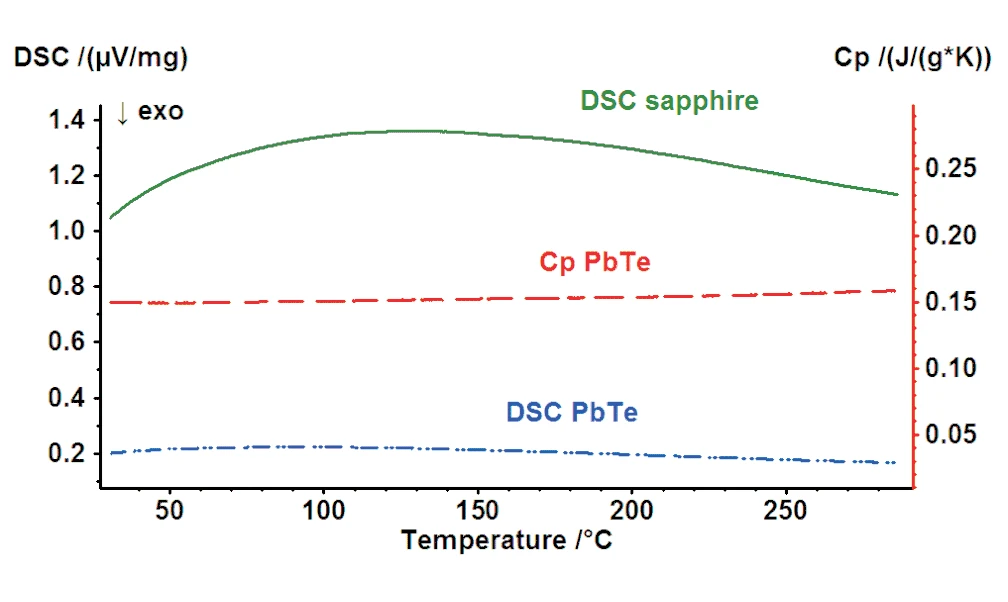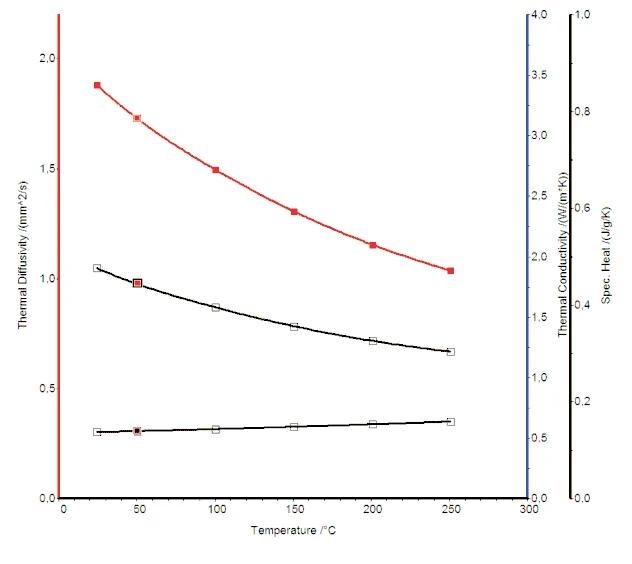# PbTe – A Thermoelectric Material Heat Capacity and Thermal Conductivity

• Thermoelectric
• LFA
• LFA 457 MicroFlash®
• DSC
• DSC 204 F1

#### Introduction

Thermoelectric substances are promising materials for waste heat harvesting. Examples of this would be the generation of electric energy by converting the heat of automobile exhaust, or from cooling devices used in power plants. Important physical properties to consider are the so-called Seebeck coefficient (S) and Conductivité ThermiqueThermal conductivity (λ with the unit W/(m•K)) describes the transport of energy – in the form of heat – through a body of mass as the result of a temperature gradient (see fig. 1). According to the second law of thermodynamics, heat always flows in the direction of the lower temperature.thermal conductivity δ). The well-known figure of merit (Z) describes the efficiency of such materials:

ZT = (S2 λ-1

with

σ= density
T = temperature

From the formula, it can be concluded that for a high Z value, the material should have a high Seebeck effect and a low thermal conductivity value.

PbTe is a potential candidate for such applications as it has a moderate Seebeck coefficient and a relatively low thermal conductivity1) Heat capacity (Specific Heat Capacity (cp)Heat capacity is a material-specific physical quantity, determined by the amount of heat supplied to specimen, divided by the resulting temperature increase. The specific heat capacity is related to a unit mass of the specimen.cp) values for PbTe

#### Results and Discussion

The specific heat of PbTe samples were measured with the NETZSCH DSC 204 F1 Phoenix® in the temperature range from RT to 300°C using the ratio method.

Figure 1 depicts the cp curve of the PbTe sample. The specific heat values are in the range of 0.15 J/(g*K) to 0.16 J/(g*K) which is typical for this material.

The Diffusivité ThermiqueThermal diffusivity (a with the unit mm2/s) is a material-specific property for characterizing unsteady heat conduction. This value describes how quickly a material reacts to a change in temperature.thermal diffusivity of PbTe was measured with the NETZSCH LFA 457 MicroFlash®. The thermal conductivity can be calculated by applying the following formula:

λ(T) = a(T) ∙ cp(T) ∙ ρ(T)

with

cp = specific heat
ρ = density
λ = thermal diffusivity

Figure 2 shows the thermal diffusivity, specific heat and thermal conductivity curves.2) Thermal conductivity, thermal diffusivity and specific heat curves of PbTe

#### Summary

The thermal diffusivity and specific heat of PbTe were measured with LFA and DSC instrumentation, respectively. The thermal conductivity, which is a very important physical property for the evaluation of efficiency of thermoelectric materials, was calculated utilizing this data along with the density of the material. The PbTe showed the expected decrease in thermal con-ductivity with increasing temperature as is normally observed for other semiconducting materials.